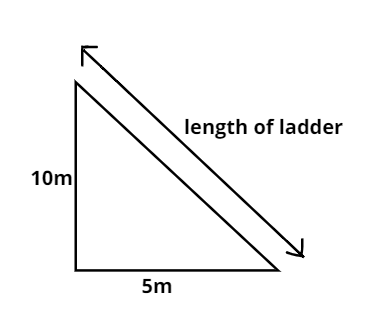Courses
Courses for Kids
Free study material
Free LIVE classes
MoreLIVE
Join Vedantu’s FREE Mastercalss

# A ladder is at a distance of 5m from the foot of the wall and it touches a window which is at a height of 10m from the ground. Find the length of the ladder.Verified
364.2k+ views
Hint- This question can be solved by using Pythagoras theorem.To solve this question let us assume a right angled triangle such that the perpendicular of the right angled triangle be the height from the ground to the window.
Height = 10m
Also, Let the base of the right angled triangle be the distance from the foot of the ladder to the wall.
$\Rightarrow$ Base$= 5m$
Hence, the hypotenuse will be the length of the ladder.
Now By Pythagoras theorem,
${\text{hy}}{{\text{p}}^2}{\text{ = bas}}{{\text{e}}^2} + {\text{heigh}}{{\text{t}}^2} \\ {\text{or hy}}{{\text{p}}^2} = {5^2} + {10^2} \\ {\text{or hy}}{{\text{p}}^2} = 25 + 100 \\ {\text{or hy}}{{\text{p}}^2} = 125 \\ {\text{or hyp = }}\sqrt {125} \\ {\text{or hyp = 5}}\sqrt 5 m \\$
Therefore, the length of the ladder is $5\sqrt 5 m$.

Note- Whenever we face such types of questions the key concept is that we should make the figure first and mention whatever is given to us and then use Pythagoras theorem, like we did in the above question.
Last updated date: 22nd Sep 2023
Total views: 364.2k
Views today: 3.64k